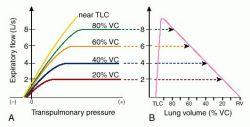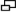# curve

(redirected from Rectifiable path)
Also found in: Dictionary, Thesaurus, Encyclopedia.
Related to Rectifiable path: Rectifiable curve

## curve

[kerv]
a line that is not straight, or that describes part of a circle, especially a line representing varying values in a graph.
dose-effect curve (dose-response curve) a graphic representation of the effect caused by an agent (such as a drug or radiation) plotted against the dose, showing the relationship of the effect to changes in the dose.
growth curve the curve obtained by plotting increase in size or numbers against the elapsed time.
oxyhemoglobin dissociation curve a graphic curve representing the normal variation in the amount of oxygen that combines with hemoglobin as a function of the partial pressures of oxygen and carbon dioxide. The curve is said to shift to the right when less than a normal amount of oxygen is taken up by the blood at a given Po2, and to shift to the left when more than a normal amount is taken up. Factors influencing the shape of the curve include changes in the blood pH, Pco2, and temperature; the presence of carbon monoxide; alterations in the constituents of the erythrocytes; and certain disease states.
pulse curve sphygmogram.
Spee curve (curve of Spee) the anatomic curvature of the occlusal alignment of teeth, beginning at the tip of the lower canine, following the buccal cusps of the premolars and molars, and continuing to the anterior border of the ramus.
strength-duration curve a graphic representation of the relationship between the intensity of an electric stimulus at the motor point of a muscle and the length of time it must flow to elicit a minimal contraction; see also chronaxie and rheobase. In cardiac pacing it is useful in determining characteristics of a particular pacing electrode and determining the most efficient selection of pacing parameters for an appropriate safety margin.
survival curve a graph of the probability of survival versus time, commonly used to present the results of clinical trials, e.g., a graph of the fraction of patients surviving (until death, relapse, or some other defined endpoint) at each time after a certain therapeutic procedure.

## curve

(kerv),
1. A nonangular continuous bend or line.
2. A chart or graphic representation, by means of a continuous line connecting individual observations, of the course of a physiologic activity, of the number of cases of a disease in a given period, or of any entity that might be otherwise presented by a table of figures. Synonym(s): chart (2)
[L. curvo, to bend]

## curve

(kerv) a line which is not straight, or which describes part of a circle, especially a line that represents varying values in a graph.Series of isovolume pressure-flow curves (A), from which can be constructed a maximal expiratory flow-volume curve (B).

Barnes curve  the segment of a circle whose center is the sacral promontory, its concavity being directed posteriorly.
curve of Carus  the normal axis of the pelvic outlet.
dental curve  c. of occlusion.
dye dilution curve  an indicator dilution curve in which the indicator is a dye, usually indocyanine green.
growth curve  the curve obtained by plotting increase in size or numbers against the elapsed time.
indicator dilution curve  a graphic representation of the concentration of an indicator added in known quantity to the circulatory system and measured over time; used in studies of cardiovascular function.
isodose curves  lines delimiting body areas receiving the same quantity of radiation in radiotherapy.
curve of occlusion  the curve of a dentition on which the occlusal surfaces lie.
oxygen-hemoglobin dissociation curve  a graphic curve representing the normal variation in the amount of oxygen that combines with hemoglobin as a function of the tension of oxygen and carbon dioxide.
Price-Jones curve  a graphic curve representing the variation in the size of the red blood corpuscles.
Starling curve  a graphic representation of cardiac output or other measure of ventricular performance as a function of ventricular filling for a given level of contractility.
strength-duration curve  a graphic representation of the relationship between the intensity of an electric stimulus at the motor point of a muscle and the length of time it must flow to elicit a minimal contraction.
temperature curve  a graphic tracing showing the variations in body temperature.
tension curves  lines observed in cancellous tissue of bones, determined by the exertion of stress during development.
ventricular function curve  Starling c.

## curve

Etymology: L, curvare, to bend
(in statistics) a straight or curved line used as a graphic method of demonstrating the distribution of data collected in a study or survey.
A nonangular deviation from a straight course in a line or surface

## curve

(kŭrv)
1. A nonangular continuous bend or line.
2. A chart or graphic representation, by means of a continuous line connecting individual observations of the course of a physiologic activity, of the number of cases of a disease in a given period, or of any entity that might be otherwise presented by a table of figures.
Synonym(s): chart (2) .
[L. curvo, to bend]

## curve

(kŭrv)
1. A nonangular continuous bend or line.
2. A chart or graphic representation, by means of a continuous line connecting individual observations, of the course of a physiologic activity, of the number of cases of a disease in a given period, or of any entity that might be otherwise presented by a table of figures.
[L. curvo, to bend]

## curve,

n a nonangular deviation from a straight line or surface.
curve, alignment,
curve, anti-Monson,
n.pr See curve, reverse.
curve, compensating,
n the curvature of alignment of the occlusal surfaces of the teeth that is developed to compensate for the paths of the condyles as the mandible moves from centric to eccentric positions. A means of maintaining posterior tooth contacts on the molar teeth and providing balancing contacts on dentures when the mandible is protruded. Corresponds to the curve of Spee of natural teeth.
curve, dose-effect,
n a curve relating the dose of radiation with the effect produced.
curve, dose-response,
n a graphical representation of the relationship between dosage (x-axis) and degree of response (y-axis); used to determine the effective dose of any given drug.
curve, milled-in,
n See path, milled-in.
curve, Monson
n.pr the curve of occlusion, described by Monson, in which each cusp and incisal edge touch or conform to a segment of the surface of a sphere 8 inches (20 cm) in diameter, with its center in the region of the glabella. See also curve, compensating.
curve of occlusion (occlusal curvature),
n 1. a curved occlusal surface that makes simultaneous contact with the major portion of the incisal and occlusal prominences of the existing teeth.
2. the curve of a dentition on which the occlusal surfaces of the teeth lie. See also curve, reverse.
curve of Spee,
n.pr 1. an anatomic curvature of the occlusal alignment of teeth, beginning at the tip of the mandibular canine, following the buccal cusps of the natural premolars and molars, and continuing to the anterior border of the ramus, as described by von Spee.
2. the curve of the occlusal surfaces of the arches in vertical dimension, brought about by a dipping downward of the mandibular premolars, with a corresponding adjustment of the maxillary premolars.
curve of Wilson,
n.pr the curvature of the cusps, as seen from the front view. The curve in the mandibular arch is concave, whereas the one in the maxillary arch is convex.
curve, reverse,
n a curve of occlusion that is convex upward when viewed in the frontal plane.
curve, sine,
n the wave form of an alternating current, characterized by a rise from zero to maximum positive potential, then descending below zero to its maximum negative value, and then rising to its maximum positive potential, to fall to zero again.
curve, survival,
n a curve obtained by plotting the number or percentage of organisms surviving at a given time against a given dose of radiation. A curve showing the percentage of individuals surviving at different intervals after a particular dosage of radiation.

## curve

a line that is not straight; the line representing varying values in a graph.

area under moment curve
plasma drug concentration × time after dosing versus time after drug administration.
area under the curve
the area under a plasma concentration versus time curve. A measure of drug absorption.
epidemic curve
a graphical representation showing the number of new cases of the disease plotted against time. A decision on when the new infection rate creates an epidemic varies with the disease and the circumstances. The rate would need to be clearly in excess of its expected frequency.
fitted curve
the theoretical frequency distribution whose closeness of fit to the subject data is under test.
freehand curve
a line drawn in by hand on a scattergram to establish the relationship between two variables.
frequency curve
a curve representing graphically the probabilities of different numbers of occurrences of an event.
logarithmic curve
the curve which demonstrates the straightline relationship between two variables when both of them are scaled as logarithms.
log dose-response curve
the standard way of presenting pharmacological data about a drug. The response is plotted against dose on a semilogarithmic graph. It has the advantage that a wide range of dose rates can be entered on the one graph.
plasma concentration-time curve
the plasma concentration of a drug plotted against time.
semilogarithmic curve
as for logarithmic curve except that only one of the variables is scaled as a logarithm. See also logarithmic relationship.
sigmoid curve
an S-shaped curve. A common curve in biological distributions.
survivorship curve
a graphic presentation of a life table. Obviously the proportion of survivors decreases with advancing age of the group.
vertebral curve
the downward curve of the thoracolumbar region and that of the cervical region in some animals.

## Patient discussion about curve

Q. I broke my pinkie finger a year ago. It is locked in a curved position. How can I straiten it out?

A. i would let a certified orthopedic look at the finger. treatment is according to the severity of the case. i think Terrany method is about finger physiotherapy. i'm not sure this method is to reshape uneven bone healing. this is a bit different situation, bone can be reshaped, this is how an orthodontic can move teeth- by changing the bone. but it takes a few years. i would go to an orthopedic, i advise you to do the same.

More discussions about curve
Site: Follow: Share:
Open / Close